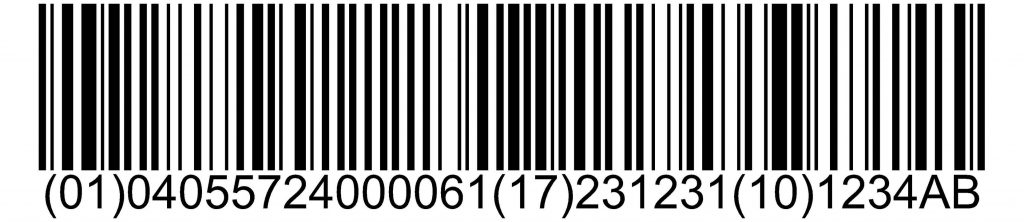10 Feb
10Feb

What is the GS1-128 label?

The EAN-128 code is used to represent the GTIN and additional information when distributing the product. That is, it is a system to identify products in the logistics environment, rather than to identify them in retail business transactions.

However, it is quite possible that you will not find information about the EAN-128 code since it is no longer called that. This code is currently known as GS1-128, as it is a standard application of GS1.What is the GS1-128 label?

A label is a piece of paper that is stuck to a product or surface to indicate any type of information, so the GS1-128 label is one that serves to represent the information of the code of the same name in order to be read .

This label must be readable both by the laser reader and by a person, which means that it must be printed, in addition to the barcode in its symbolic form and its representation in digits, the information contained in this code in a human-readable format.

What is the check digit?

The check digit is one of the integral parts of any code. Its function in the code is not the same as the rest of the digits, since it does not contain any type of information about the product.

That digit ensures the correct reading of the GTIN, eliminating errors almost entirely. This is achieved thanks to the fact that it avoids the error when performing the automatic treatment of the GTIN code.

The digit is calculated by means of a specific algorithm that guarantees its precision, as well as total security in reading the GTIN. That is, the digit plays the most important role in achieving a reliable reading.

How to calculate the EAN 128 check digit

The check digit can be easily calculated through the Check Digit Calculator | GS1 Hong Kong, thanks to the fact that it offers an application to perform the calculation automatically. However, we believe it is important to know the process, both to understand what happens in the algorithm and to be able to perform the operation manually.

First, list the twelve digits of the code from right to left.

Once a number is assigned, multiply the numbers in even position by 1 and the numbers in odd position by 3.

So, in the example code '123456789012', the 0 would be multiplied by 3 and the 9 by 1.

Once you have obtained the results of the multiplications, the results are added.

In the case of the example shown, the sum would be 1+6+3+12+5+18+7+24+9+0+1+6, with a result of 92.

In the next step, find the top ten of the result, that is, the closest multiple of 10 to the result, above.

In the case of our example, it would be 100. Now, subtract the result of the sum from the multiple of 10, and you will have your check digit.

The last step is to add this digit to the end of the code, thus obtaining the final code.

In the example shown, the final code would be: 1234567890128, since 8 is the result of subtracting 100 from 92.